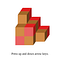# Step 1: Understanding the problem

`segments :: [Int]segments =    [2, 1, 1, 1, 2, 1, 2, 1, 1, 1, 1, 2, 1, 2, 1, 1, 1, 1, 1, 2]`
`type Pos = (Int, Int, Int)data Dir = F | B | L | R | U | D deriving (Show)move :: Dir -> Pos -> Posmove F (i, j, k) = (i + 1, j, k)move B (i, j, k) = (i - 1, j, k)move L (i, j, k) = (i, j - 1, k)move R (i, j, k) = (i, j + 1, k)move U (i, j, k) = (i, j, k + 1)move D (i, j, k) = (i, j, k - 1)`

# Step 2: Recursive tree search

`turns :: Dir -> [Dir]turns F = [L, R, U, D]turns B = [L, R, U, D]turns L = [F, B, U, D]turns R = [F, B, U, D]turns U = [F, B, L, R]turns D = [F, B, L, R]`
`data SearchState = SearchState {    currentPos :: Pos,    currentDir :: Dir,    remainingSegments :: [Int],    usedPositions :: Set Pos    }`
`initialSearchStates :: [SearchState]initialSearchStates = [    SearchState {        currentPos = (0, j, k),        currentDir = F,        remainingSegments = segments,        usedPositions = Set.empty    }    | j <- [0..2], k <- [0..2] ]`
`goodPosition :: Set Pos -> Pos -> BoolgoodPosition used pos =    inRange ((0,0,0),(2,2,2)) pos && not (pos `Set.member` used)`
`search :: SearchState -> [[Dir]]search SearchState{ remainingSegments = [] } = [[]]search SearchState{..}  | all (goodPosition usedPositions) cover        = [ currentDir : solution          | dir' <- turns currentDir          , solution <- search SearchState{              currentPos = last covered,              currentDir = dir',              remainingSegments = segs,              usedPositions = foldr Set.insert usedPositions                                    (init cover)              }          ]  | otherwise = []  where s:segs = remainingSegments        cover = take (s + 1) (iterate (move currentDir) currentPos)`
`theAnswer :: [Dir]theAnswer = head (concatMap search initialSearchStates)`

# Step 3: Visualization

`blocks :: [Pos]blocks = follow (0, 0, 0) (zip theAnswer segments)  where follow p [] = [p]        follow p ((_, 0) : steps) = follow p steps        follow p ((d, n) : steps)             = p : follow (move d p) ((d, n - 1) : steps)`
`main :: IO ()main = activityOf initial change pictureinitial :: Intinitial = 1change :: Event -> Int -> Intchange (KeyPress "Up")   n = n + 1change (KeyPress "Down") n = n - 1change other             n = n`
`picture :: Int -> Picturepicture n = pictures [    drawBlock p    | p <- sortBy (comparing viewSortKey) (take n blocks)    ]  where viewSortKey (i, j, k) = (i, -j, -k)drawBlock :: Pos -> PicturedrawBlock (i, j, k) = pictures [    -- The front face.    colored (light gray) \$ solidPolygon [        project p | p <- [ (x + 0.5, y + 0.5, z - 0.5),                           (x + 0.5, y - 0.5, z - 0.5),                           (x - 0.5, y - 0.5, z - 0.5),                           (x - 0.5, y + 0.5, z - 0.5) ] ],    -- The top face.    colored gray \$ solidPolygon [        project p | p <- [ (x + 0.5, y + 0.5, z + 0.5),                           (x + 0.5, y + 0.5, z - 0.5),                           (x - 0.5, y + 0.5, z - 0.5),                           (x - 0.5, y + 0.5, z + 0.5) ] ],    -- The right face.    colored (dark gray) \$ solidPolygon [        project p | p <- [ (x + 0.5, y + 0.5, z + 0.5),                           (x + 0.5, y + 0.5, z - 0.5),                           (x + 0.5, y - 0.5, z - 0.5),                           (x + 0.5, y - 0.5, z + 0.5) ] ]    ]  where x = fromIntegral j        y = fromIntegral k        z = fromIntegral iproject :: (Double, Double, Double) -> Pointproject (x, y, z) = (3 * x + (1 + sin t / 4) * z,                     3 * y + (1 + cos t / 4) * z)`The solver for the snake puzzle

Written by

## Chris Smith

#### Software engineer at Google, volunteer math and computer science teacher, author of the CodeWorld platform, amateur ring theorist, and Haskell enthusiast.

Welcome to a place where words matter. On Medium, smart voices and original ideas take center stage - with no ads in sight. Watch
Follow all the topics you care about, and we’ll deliver the best stories for you to your homepage and inbox. Explore
Get unlimited access to the best stories on Medium — and support writers while you’re at it. Just \$5/month. Upgrade